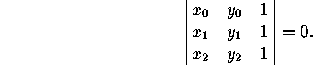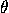Next: 5 Polygons
Up: 4 Lines
Previous: 4.2 Angles

# 4.3 Concurrence and Collinearity

Three lines ax+by+c=0, ax+by+c=0, and ax+by+c=0 are concurrent if and only if(This remains true in oblique coordinates.)

Three points (x,y), (x,y) and (x,y) are collinear if and only if(This remains true in oblique coordinates.)

Three points with polar coordinates (r,), (r,) and (r,) are collinear if and only if

rrsin(-)+ rrsin(-)+ rrsin(-)=0.

Next: 5 Polygons
Up: 4 Lines
Previous: 4.2 AnglesThe Geometry Center Home Page

Silvio Levy
Wed Oct 4 16:41:25 PDT 1995

This document is excerpted from the 30th Edition of the CRC Standard Mathematical Tables and Formulas (CRC Press). Unauthorized duplication is forbidden.